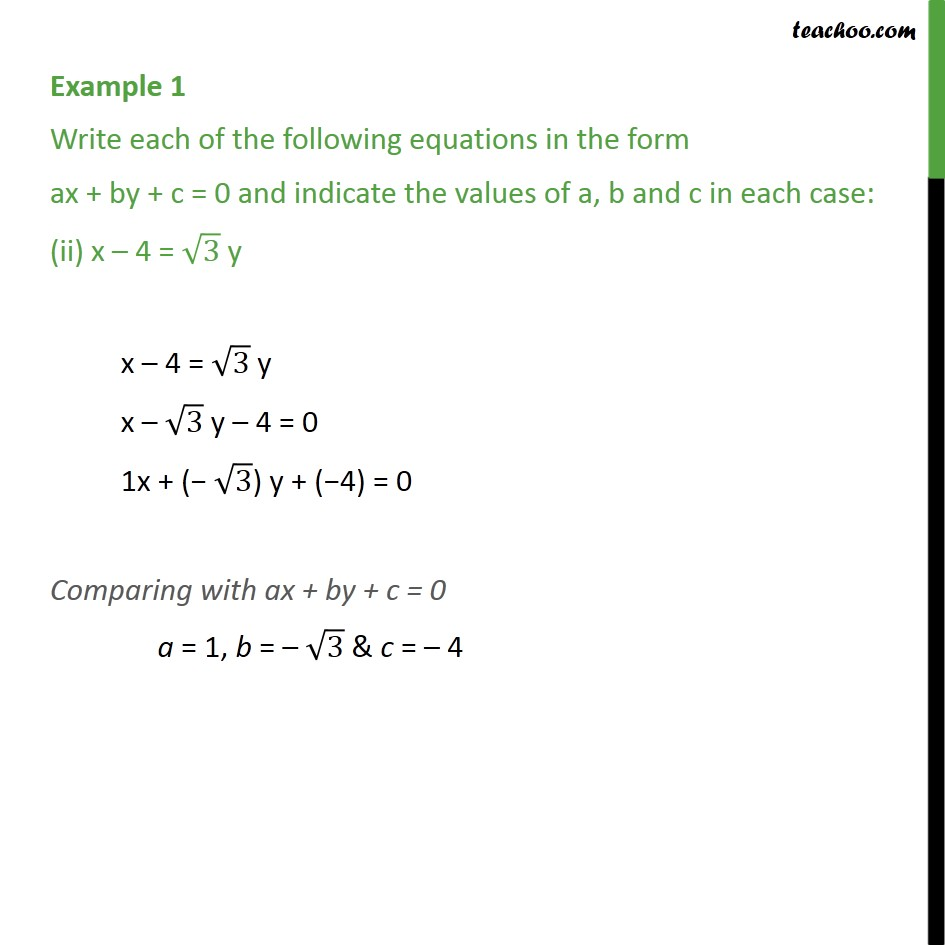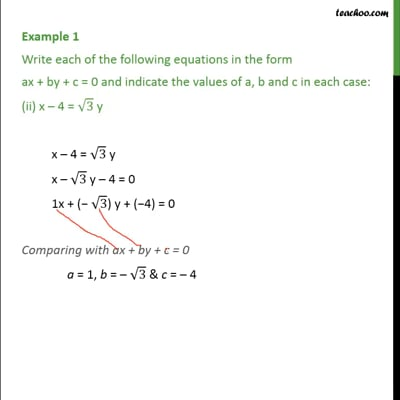Examples

Chapter 4 Class 9 Linear Equations in Two Variables
Serial order wiseThis video is only available for Teachoo black users

Learn in your speed, with individual attention - Teachoo Maths 1-on-1 Class

### Transcript

Example 1 Write each of the following equations in the form ax + by + c = 0 and indicate the values of a, b and c in each case: (ii) x – 4 = √3 y x – 4 = √3 y x – √3 y – 4 = 0 1x + (− √3) y + (−4) = 0 Comparing with ax + by + c = 0 a = 1, b = – √3 & c = – 4